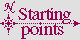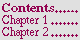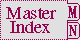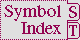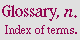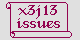#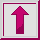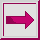Variable +, ++, +++

Value Type:

an object.

Initial Value:

Description:

The variables +, ++, and +++ are maintained by the Lisp read-eval-print loop to save forms that were recently evaluated.

The value of + is the last form that was evaluated, the value of ++ is the previous value of +, and the value of +++ is the previous value of ++.

Examples:

```(+ 0 1) =>  1
(- 4 2) =>  2
(/ 9 3) =>  3
(list + ++ +++) =>  ((/ 9 3) (- 4 2) (+ 0 1))
(setq a 1 b 2 c 3 d (list a b c)) =>  (1 2 3)
(setq a 4 b 5 c 6 d (list a b c)) =>  (4 5 6)
(list a b c) =>  (4 5 6)
(eval +++) =>  (1 2 3)
#.`(,@++ d) =>  (1 2 3 (1 2 3))
```

Affected By: